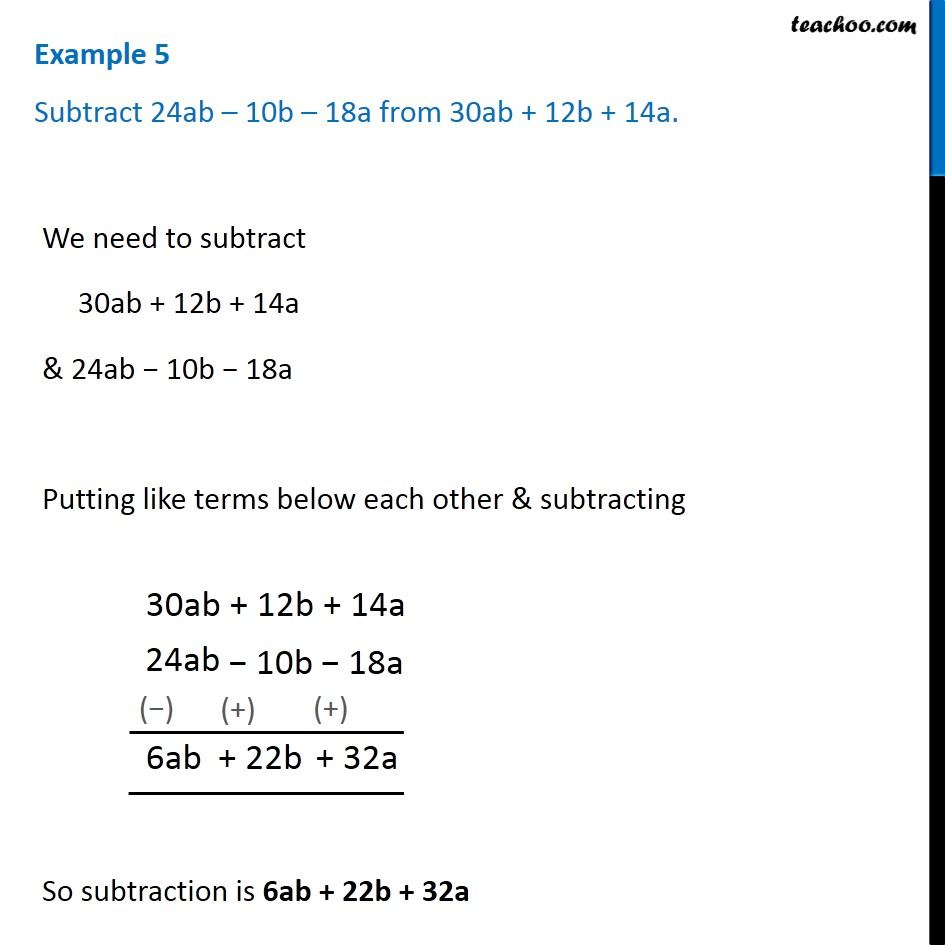1. Chapter 12 Class 7 Algebraic Expressions
2. Concept wise
3. Addition and Subtraction of Algebraic Expressions

Transcript

Example 5 Subtract 24ab – 10b – 18a from 30ab + 12b + 14a. We need to subtract 30ab + 12b + 14a & 24ab − 10b − 18a Putting like terms below each other & subtracting So subtraction is 6ab + 22b + 32a

Addition and Subtraction of Algebraic Expressions